# Propositional Logic Equivalence Laws

Boolean Algebra

In this tutorial we will cover Equivalence Laws.

## Equivalence statements

Two statements are said to be equivalent if they have the same truth value.

Example
Following are two statements.
p = It is false that he is a singer or he is a dancer.
q = He is not a singer and he is not a dancer.

The first statement p consists of negation of two simple proposition
a = He is a singer.
b = He is a dancer.
They are connected by an OR operator (connective)
so we can write,
p = ~(a ∨ b)

The second statement q consists of two simple proposition
which are negation of a and b
~a = He is not a singer.
~b = He is not a dancer.
They are connected by an AND operator (connective)
so we can write,
q = ~a ∧ ~b

We have,
p = ~(a ∨ b)
q = ~a ∧ ~b
following are the truth tables for p and q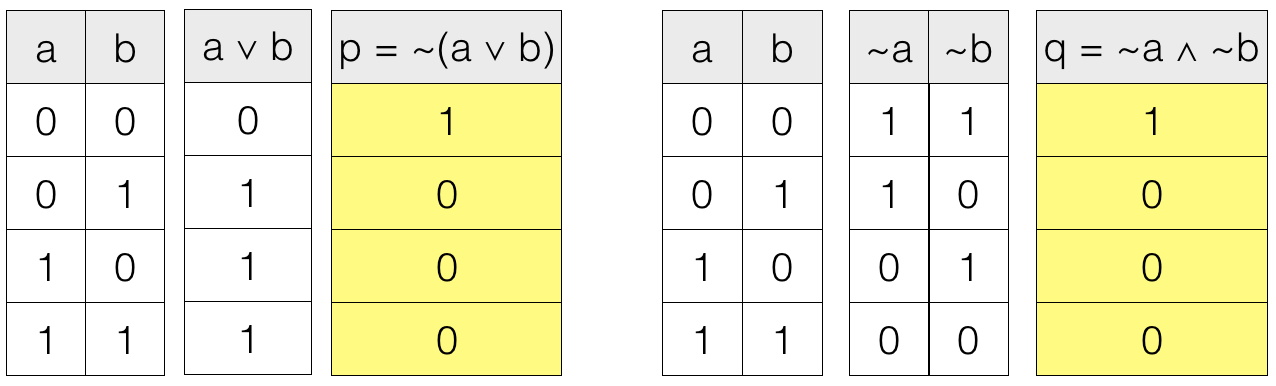We can see that the truth values are same for both the statements. So, p and q are equivalent statements.

Now we will cover some equivalence laws.

## Properties of 0

If x is a statement then,
0 + x = x
0 . x = 0
where + is the OR operator and
. is the AND operator

Truth table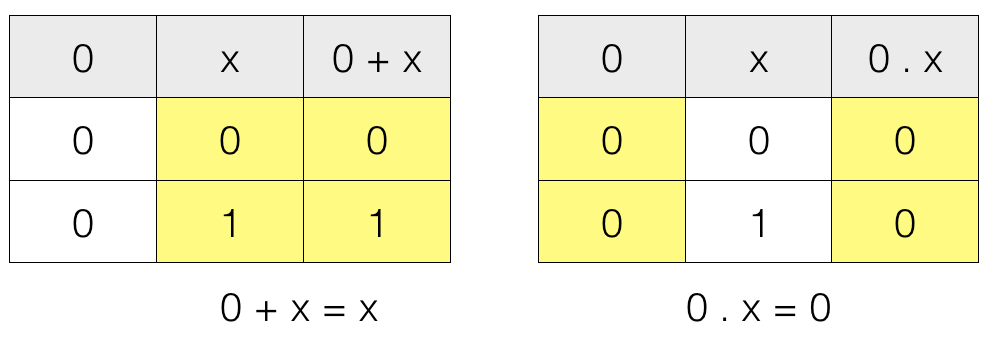## Properties of 1

If x is a statement then,
1 + x = 1
1 . x = x
where + is the OR operator and
. is the AND operator

Truth table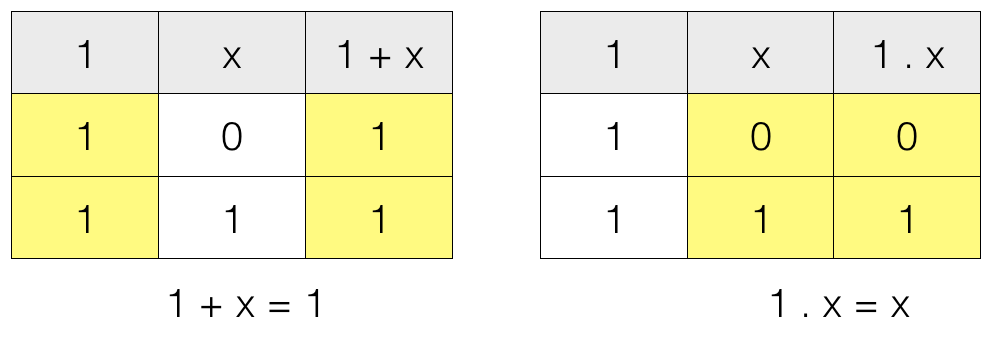## Involution

If p is a statement then,
~(~p) = p
where ~ is the NOT operator

Truth table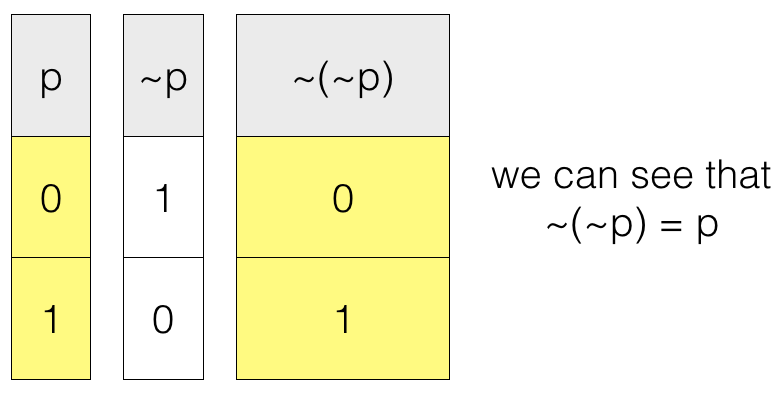## Idempotence Law

If p is a statement then,
p + p = p
p . p = p
where + is the OR operator and
. is the AND operator

Truth table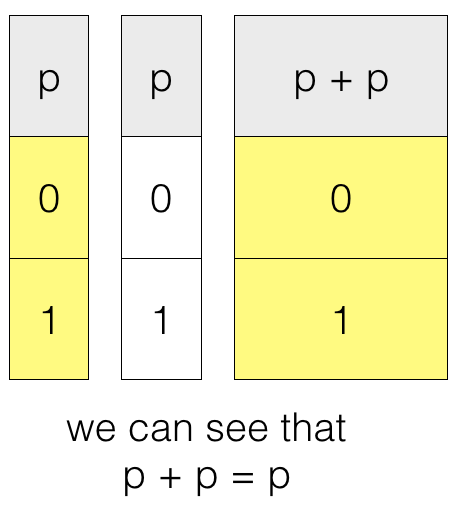## Absorption Law

If p and q are two statements then,
p + (p.q) = p
p . (p + q) = p
where + is the OR operator and
. is the AND operator

Truth table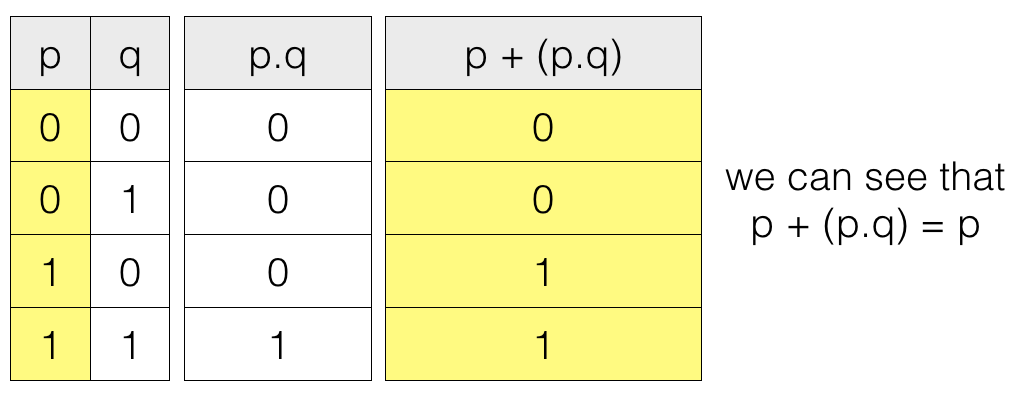## Complementarity Law

If p is a statement then,
p + (~p) = 1
p . (~p) = 0
where + is the OR operator,
. is the AND operator and
~ is the NOT operator

Truth table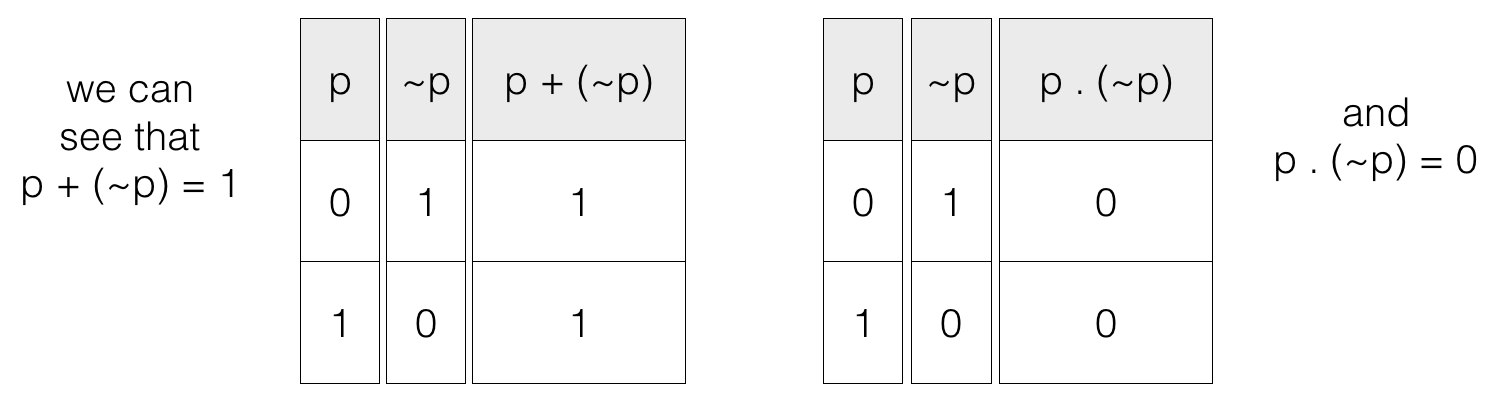## Commutative Law

If p and q are two statements then,
p + q = q + p
p . q = q . p
where + is the OR operator and
. is the AND operator

## Associative Law

If p, q and r are three statements then,
(p + q) + r = p + (q + r)
(p . q) . r = p . (q . r)
where + is the OR operator and
. is the AND operator

## Distributive Law

If p, q and r are three statements then,
p . (q + r) = (p . q) + (p . r)
p + (q . r) = (p + q) . (p + r)
p + (~p . q) = p + q
where + is the OR operator,
. is the AND operator and
~ is the NOT operator

## De Morgan's Law

If p and q are two statements then,
~(p + q) = ~p . ~q
~(p . q) = ~p + ~q
where + is the OR operator,
. is the AND operator and
~ is the NOT operator

Truth table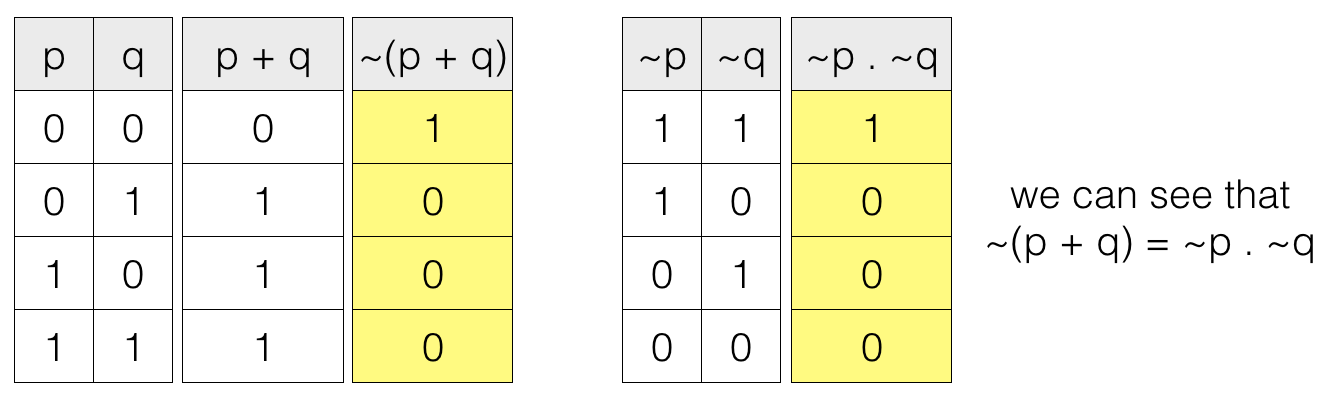## Conditional Elimination

If p and q are two statements then,
p ⇒ q = ~p + q
where + is the OR operator and
~ is the NOT operator

## Bi-conditional Elimination

If p and q are two statements then,
p ⇔ q = (p . q) + (~p . ~q)
where + is the OR operator,
. is the AND operator and
~ is the NOT operator